# MSBSHSE Solutions For SSC (Class 10) Maths Part 2 Chapter 2 - Pythagoras Theorem

MSBSHSE Solutions For SSC (Class 10) Maths Part 2 Chapter 2 – Pythagoras Theorem consists of 100% accurate solutions prepared by our experts. These solutions provide students an advantage with practical questions. Each step in the solution is explained to match students’ understanding. This chapter deals with Pythagoras theorem, Pythagorean triplet, Theorem of Geometric Mean and application of Pythagoras Theorem. These solutions help the students to enhance their knowledge in Pythagoras theorem and crack different types of problems easily. All solutions from MSBSHSE Solutions For SSC (Class 10) Maths Part 2 Chapter 2 provided here are for free.

## Download the PDF of Maharashtra Solutions For SSC Maths Part 2 Chapter 2 Pythagoras Theorem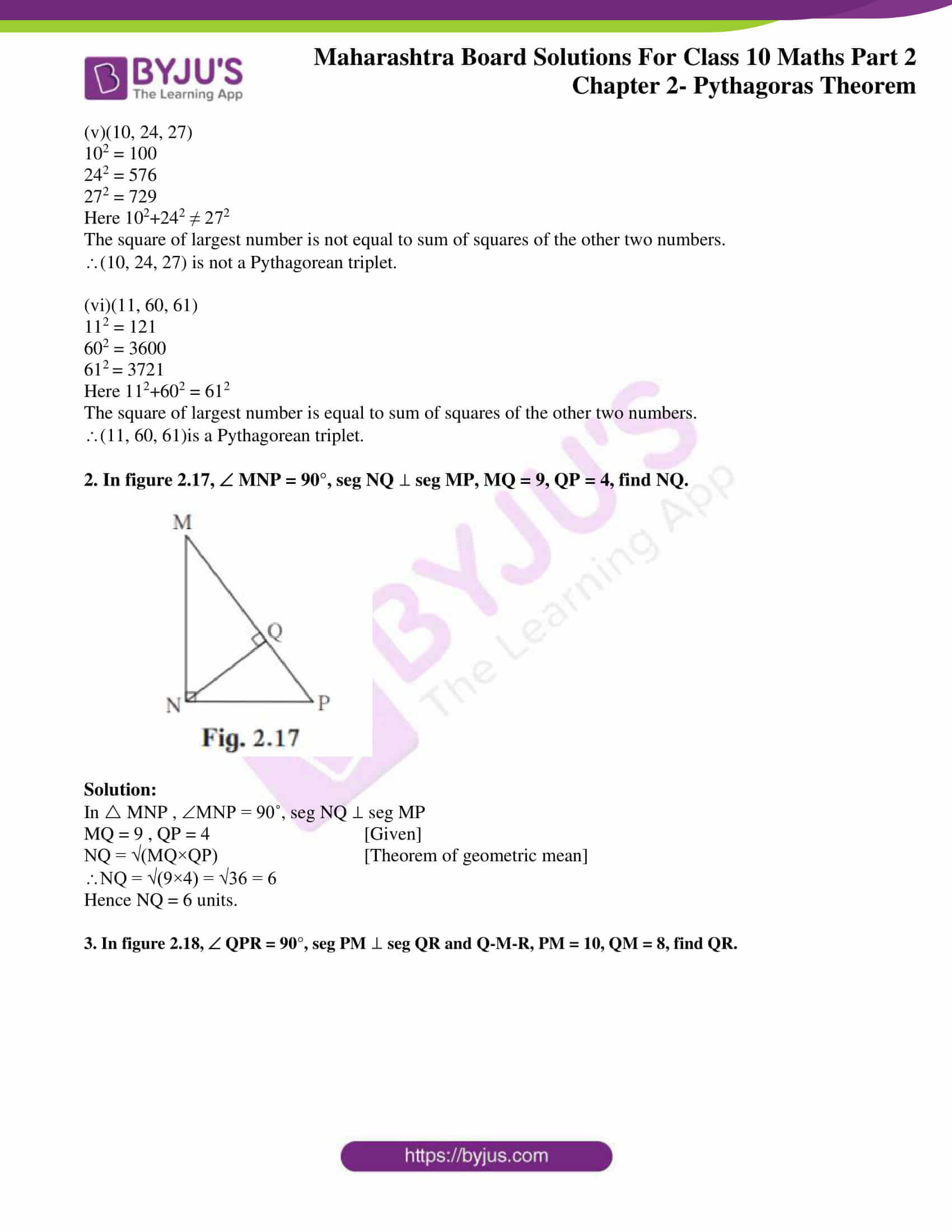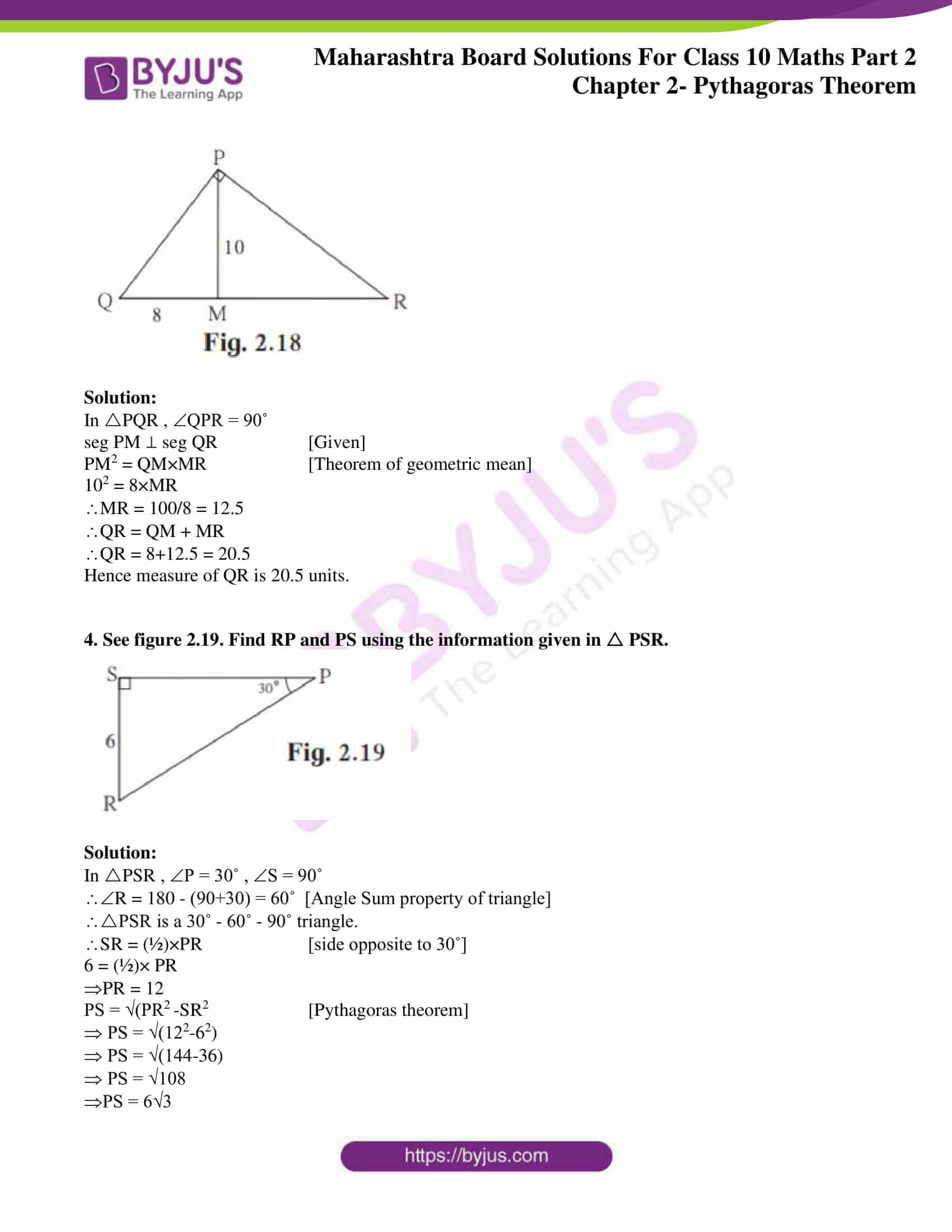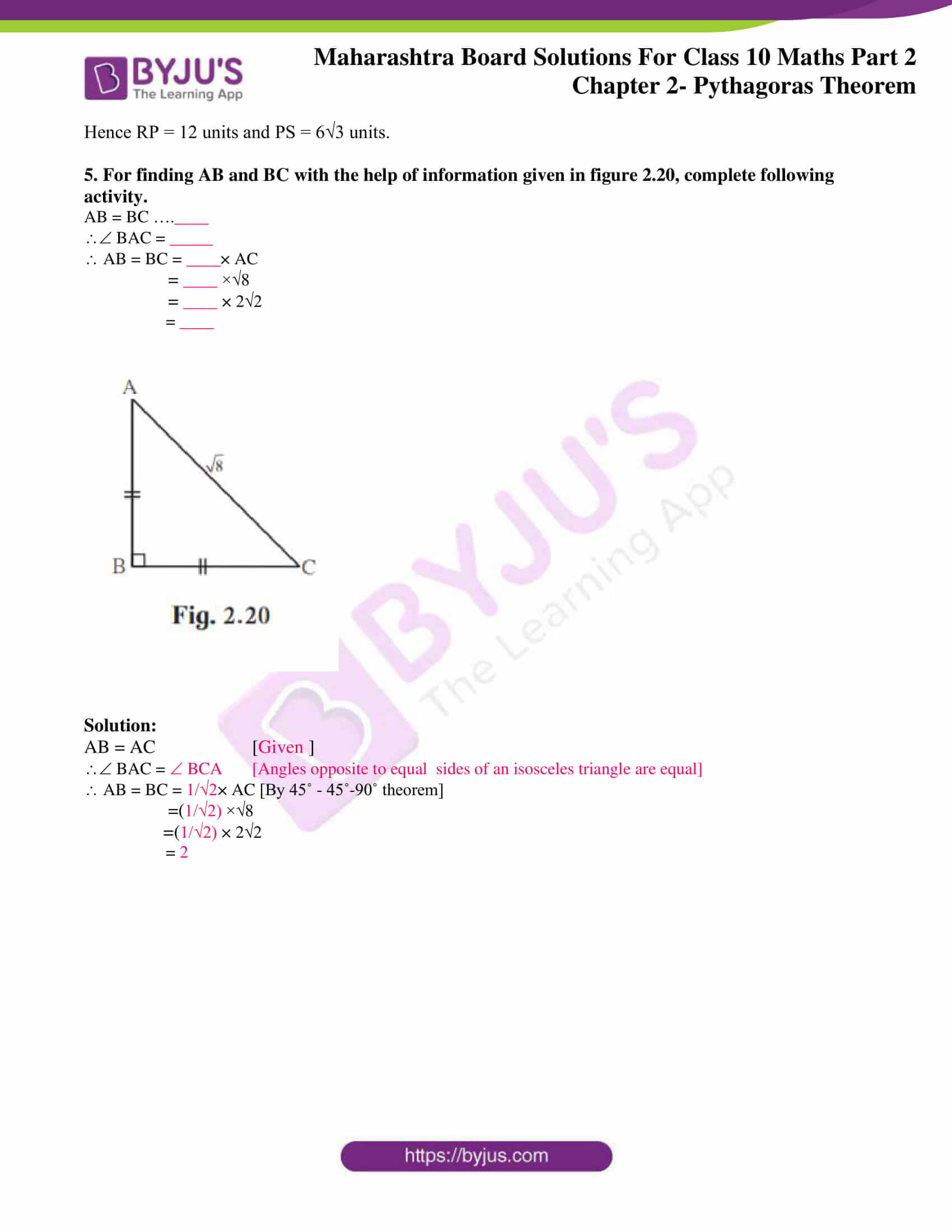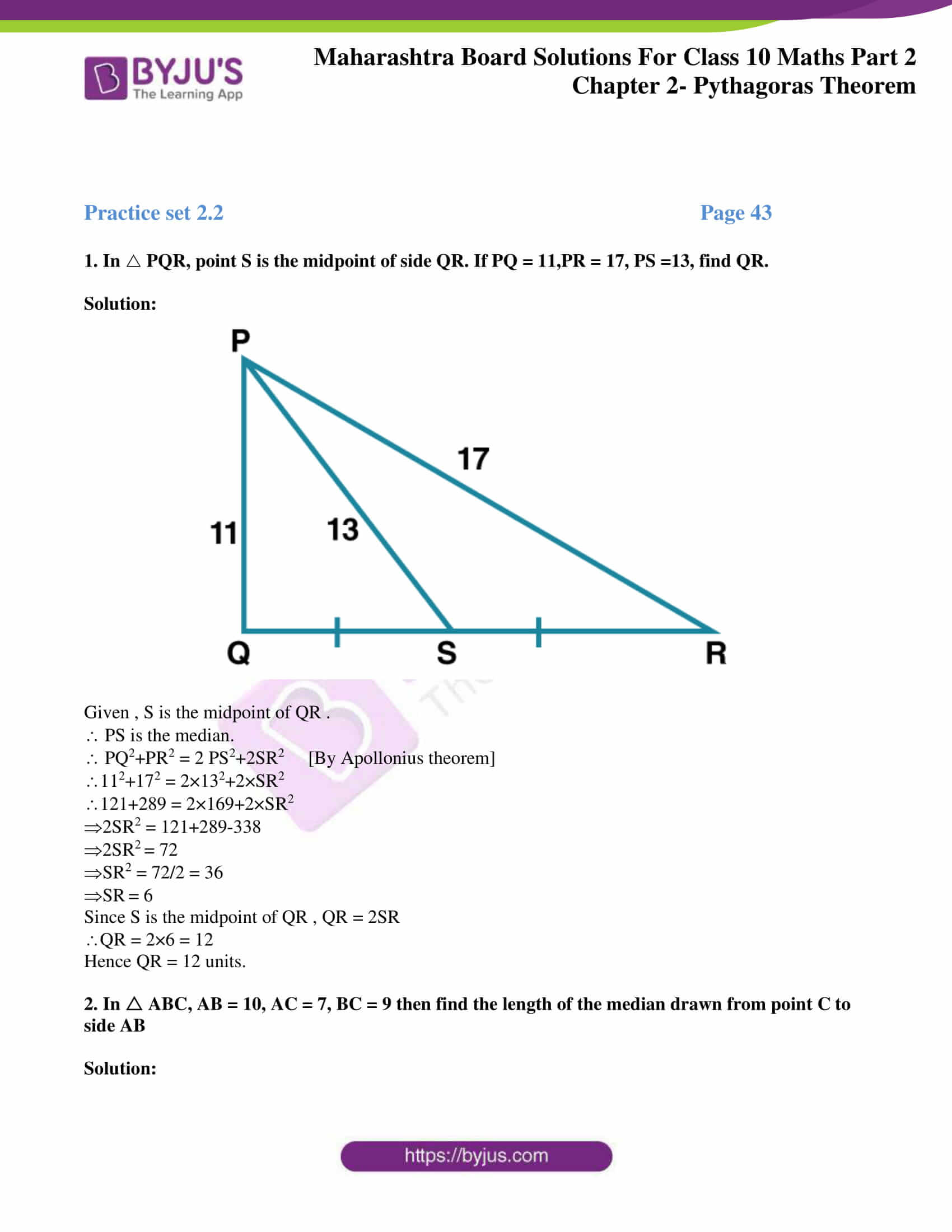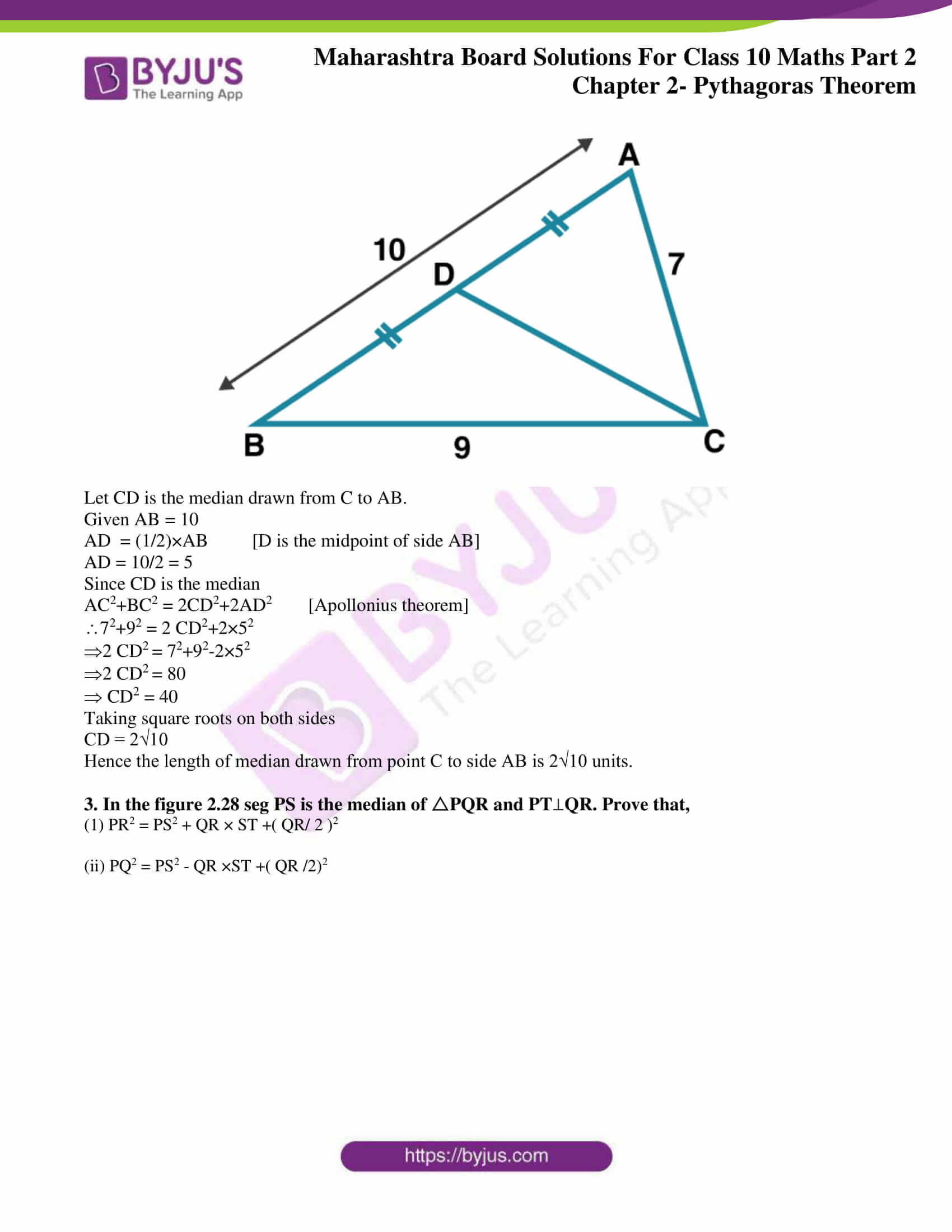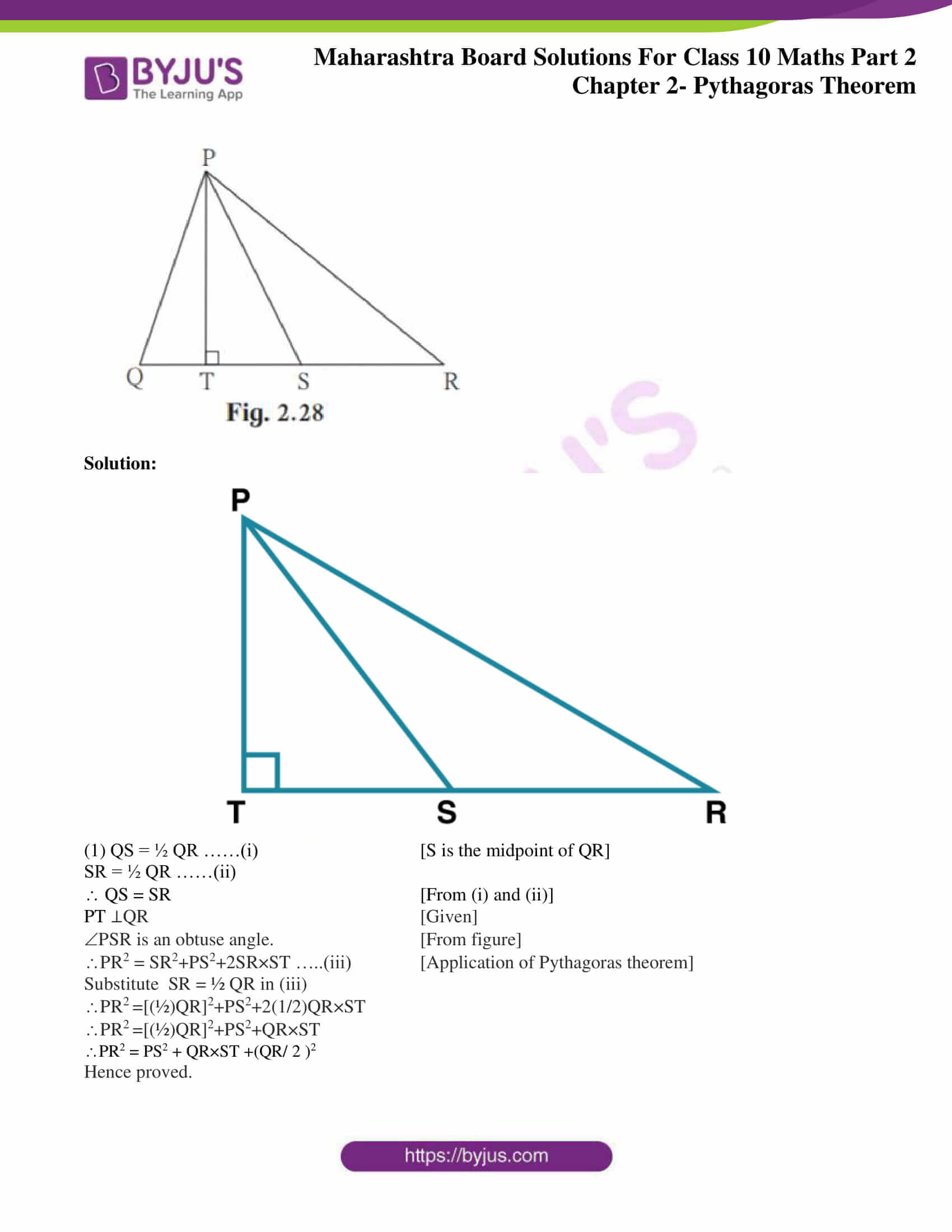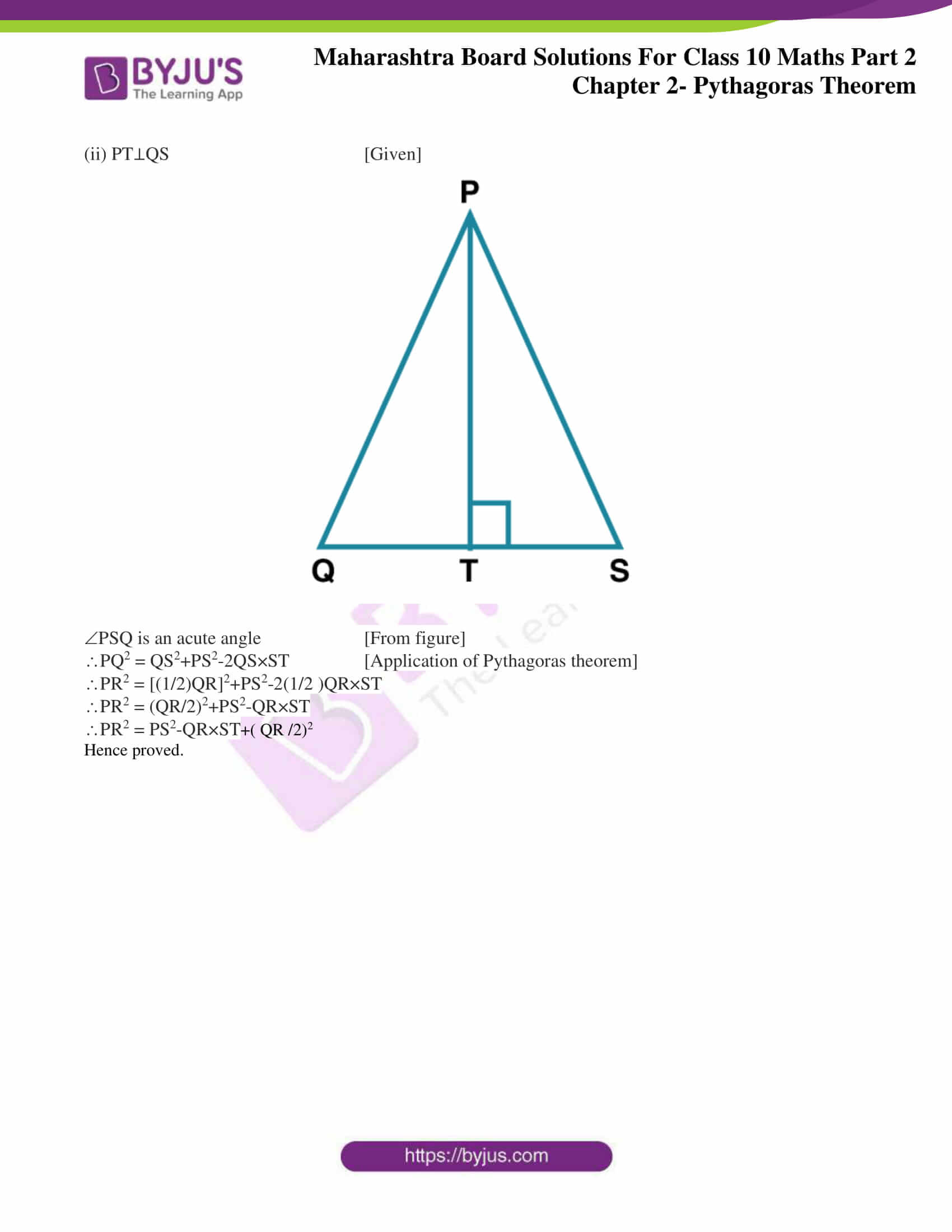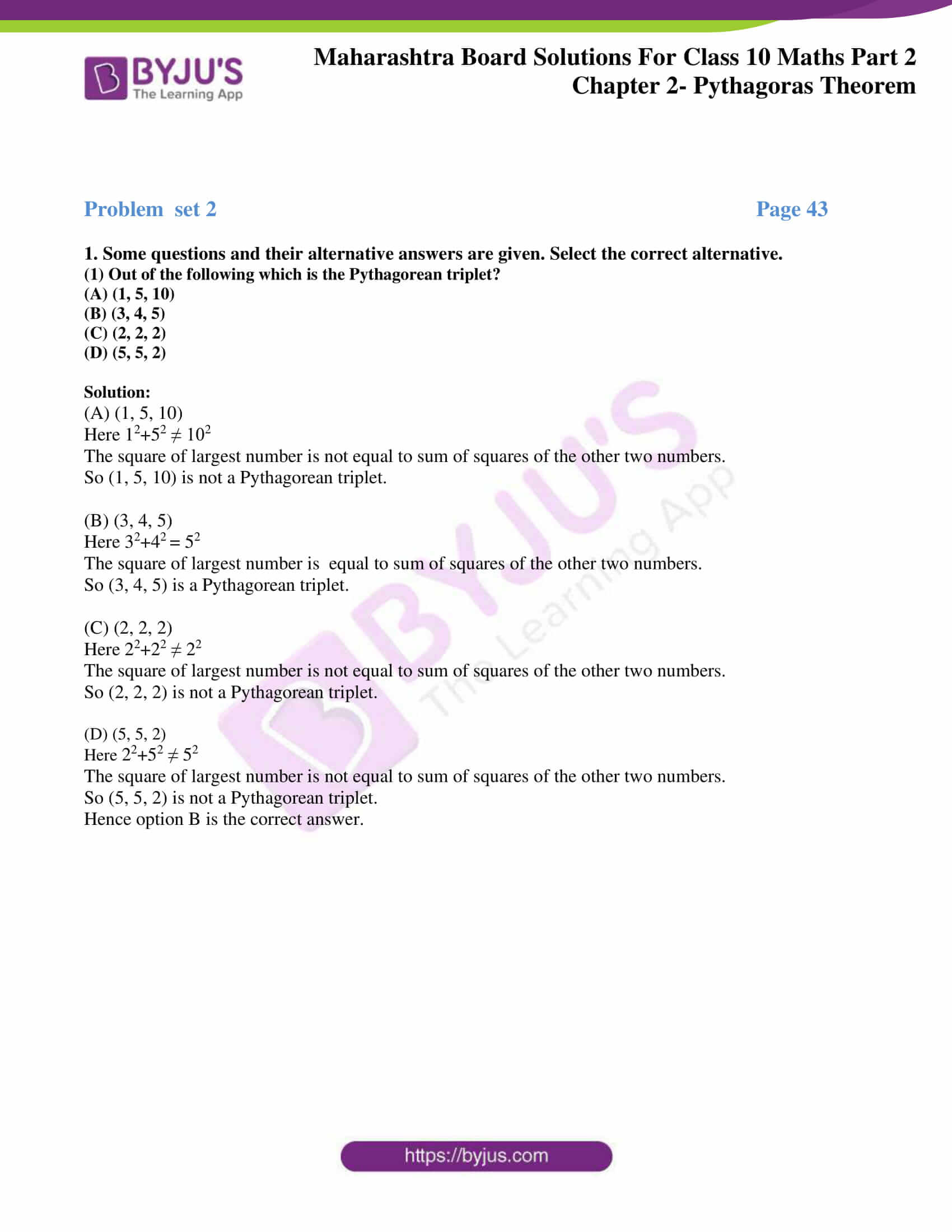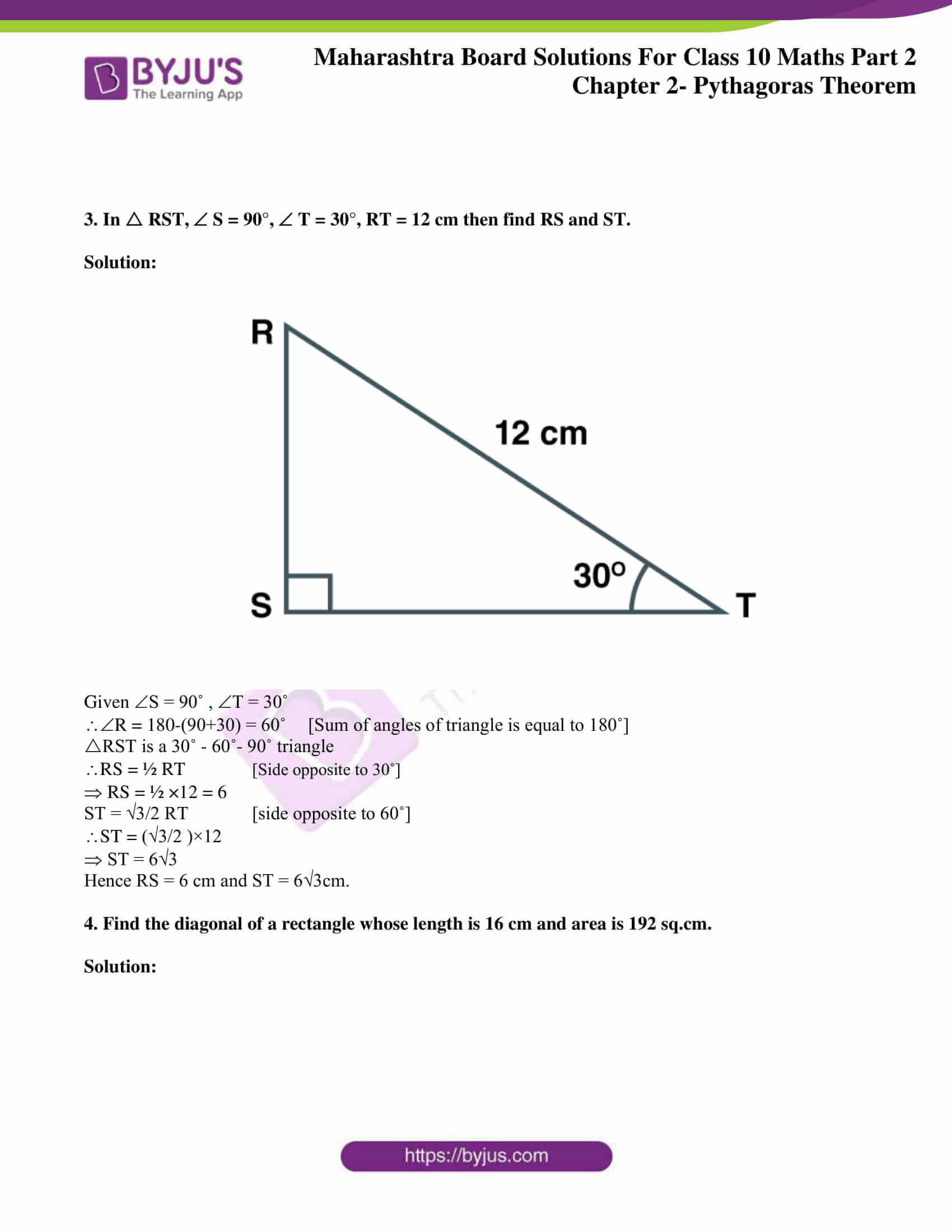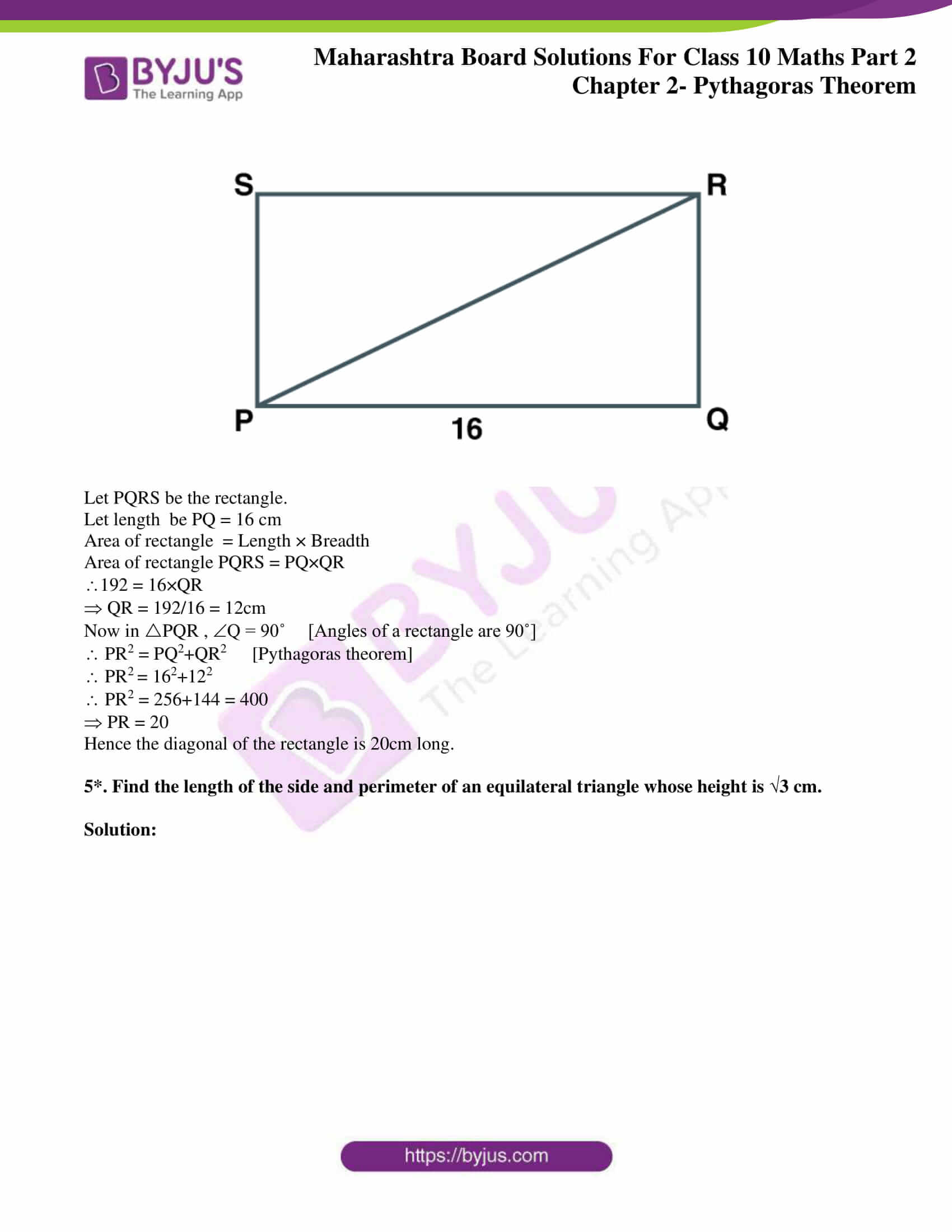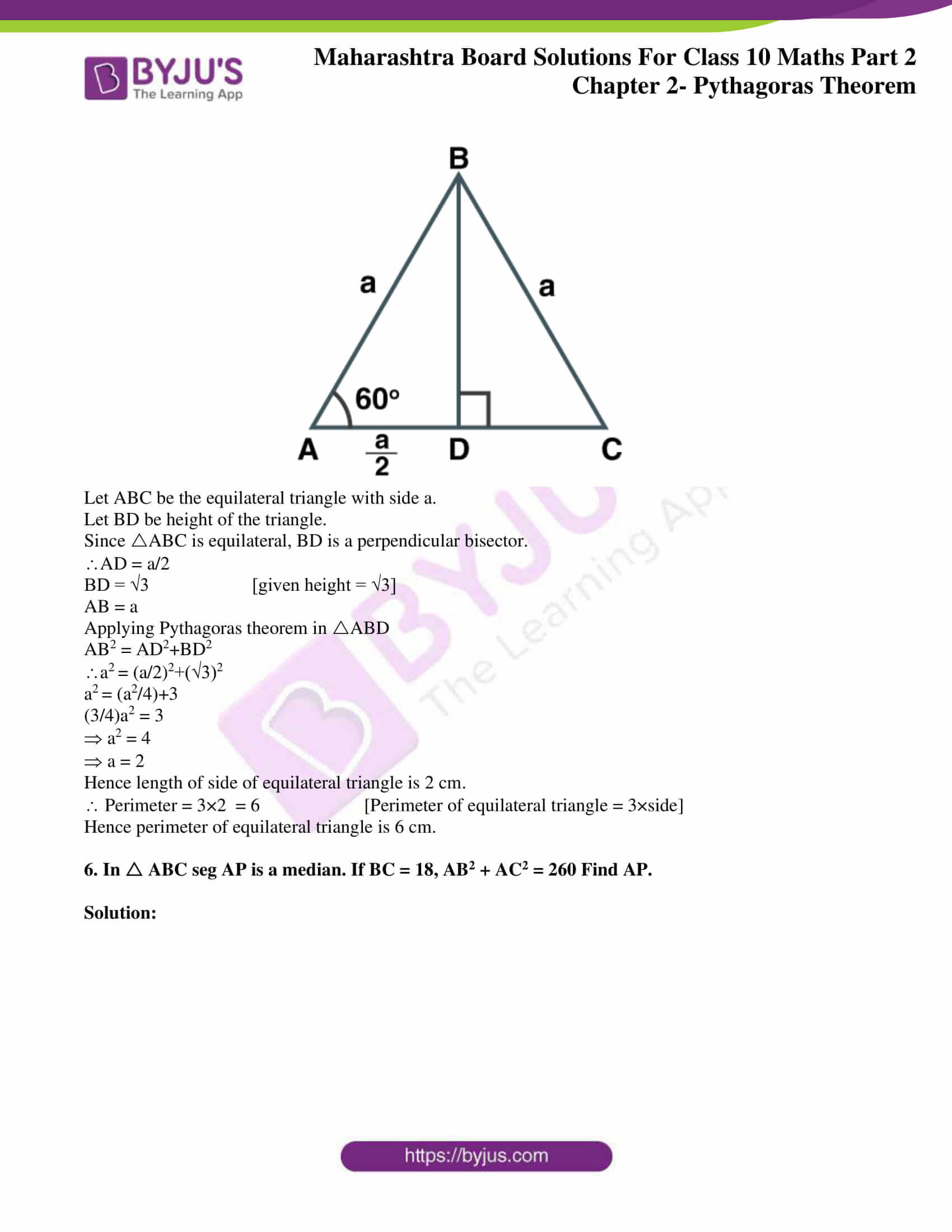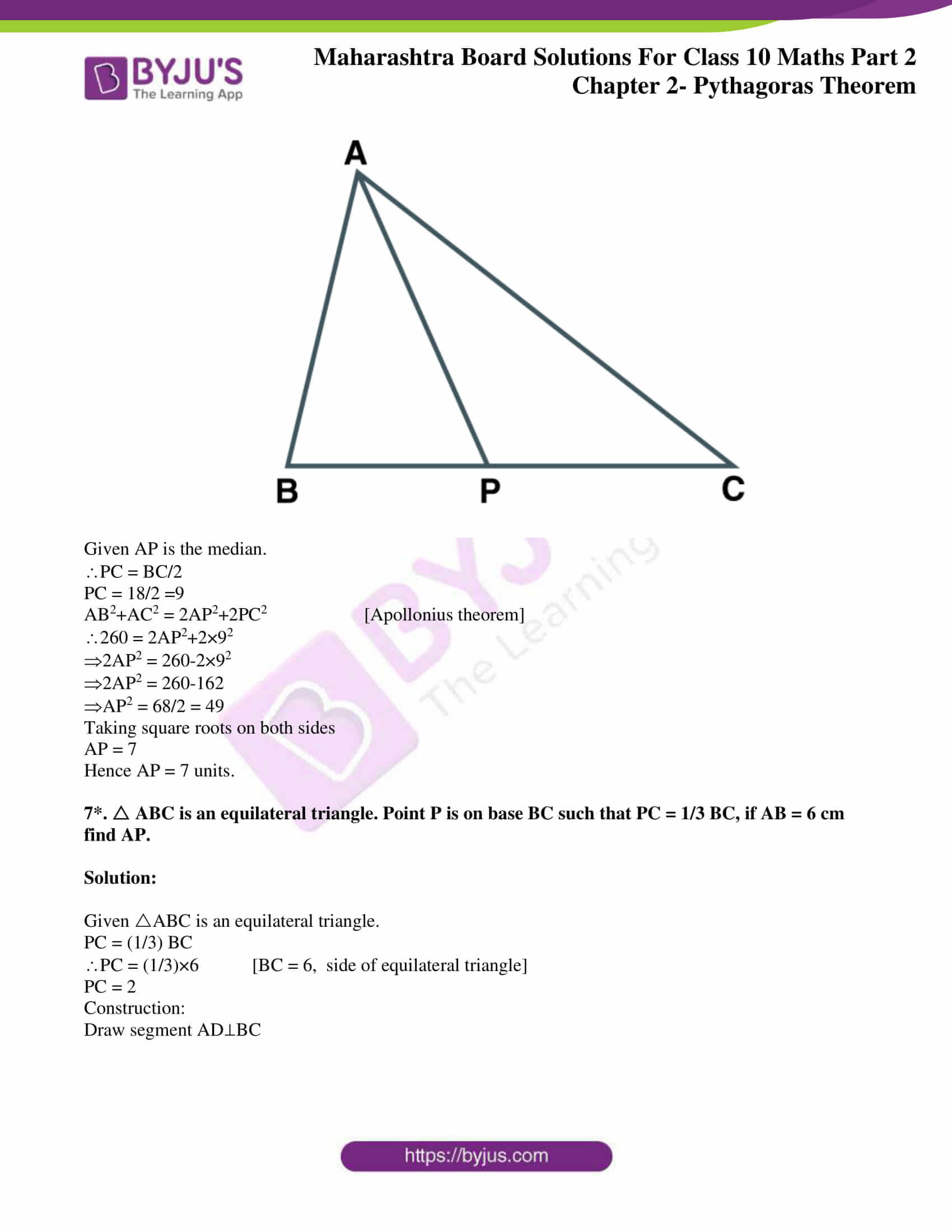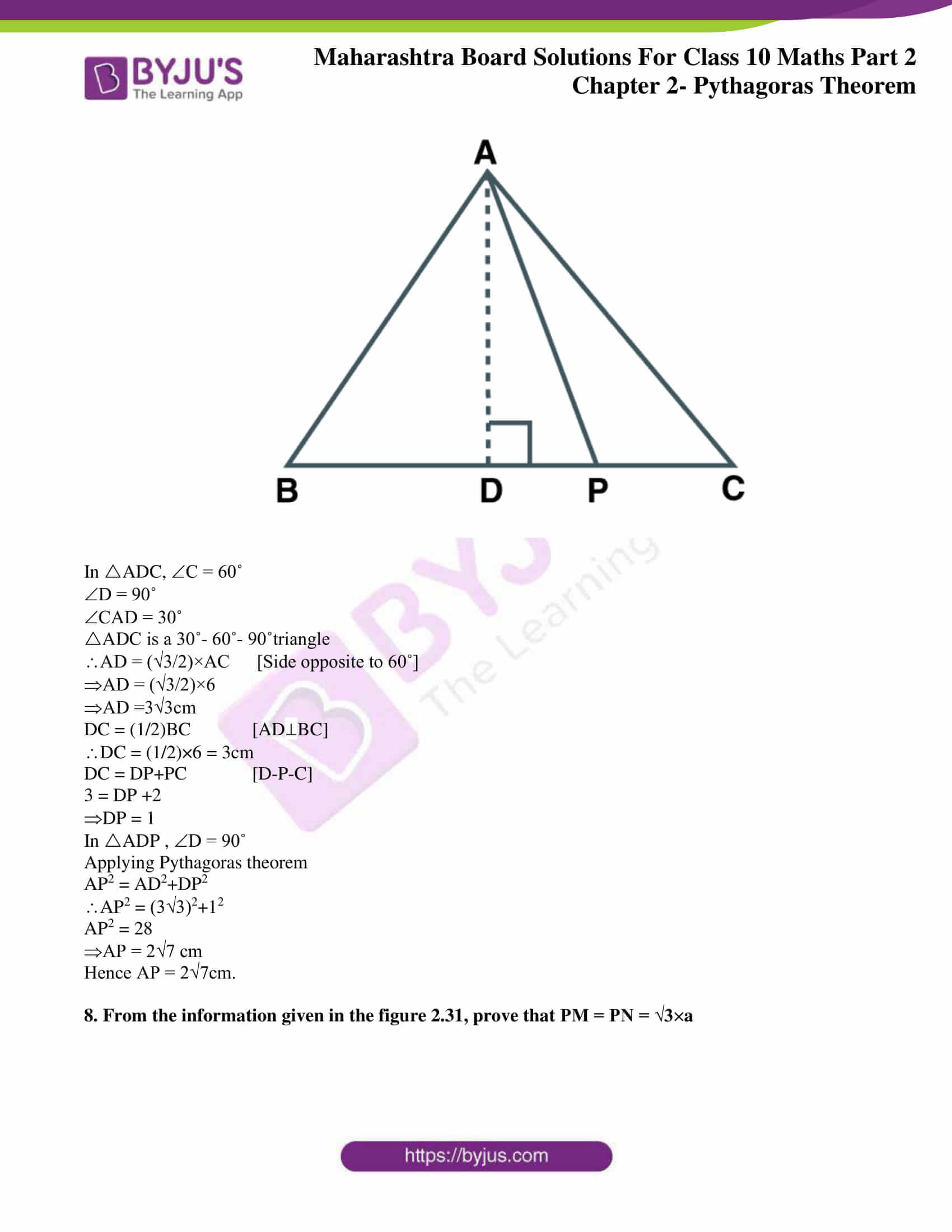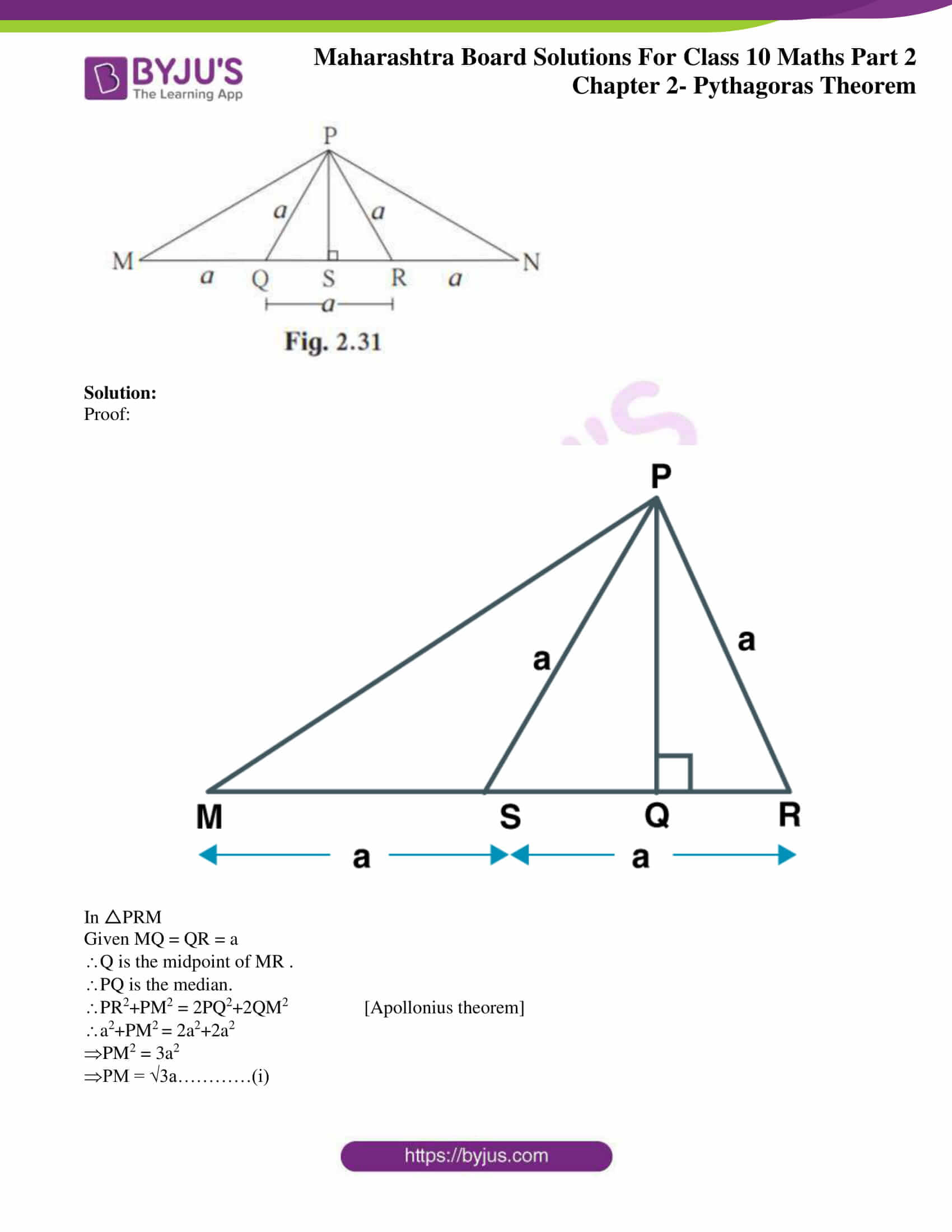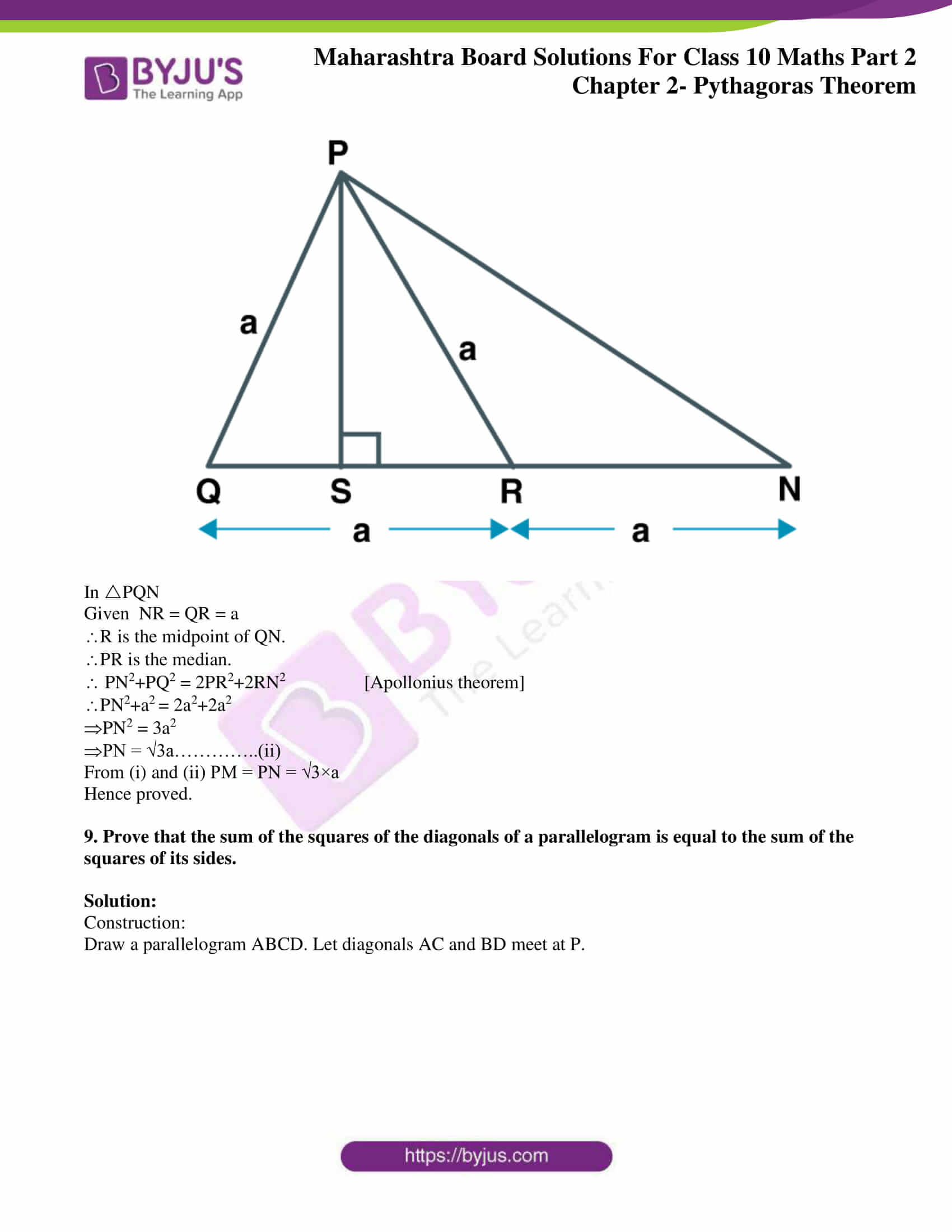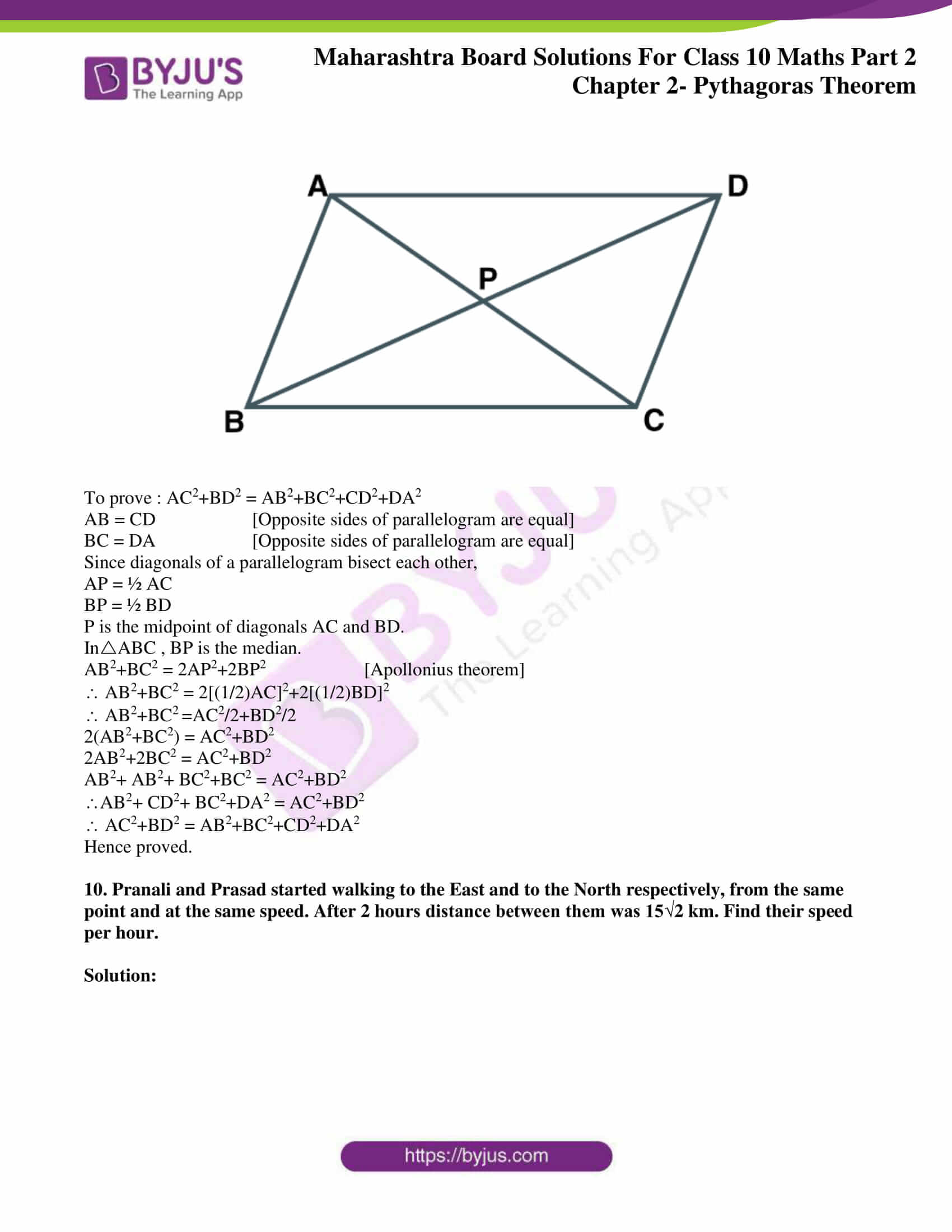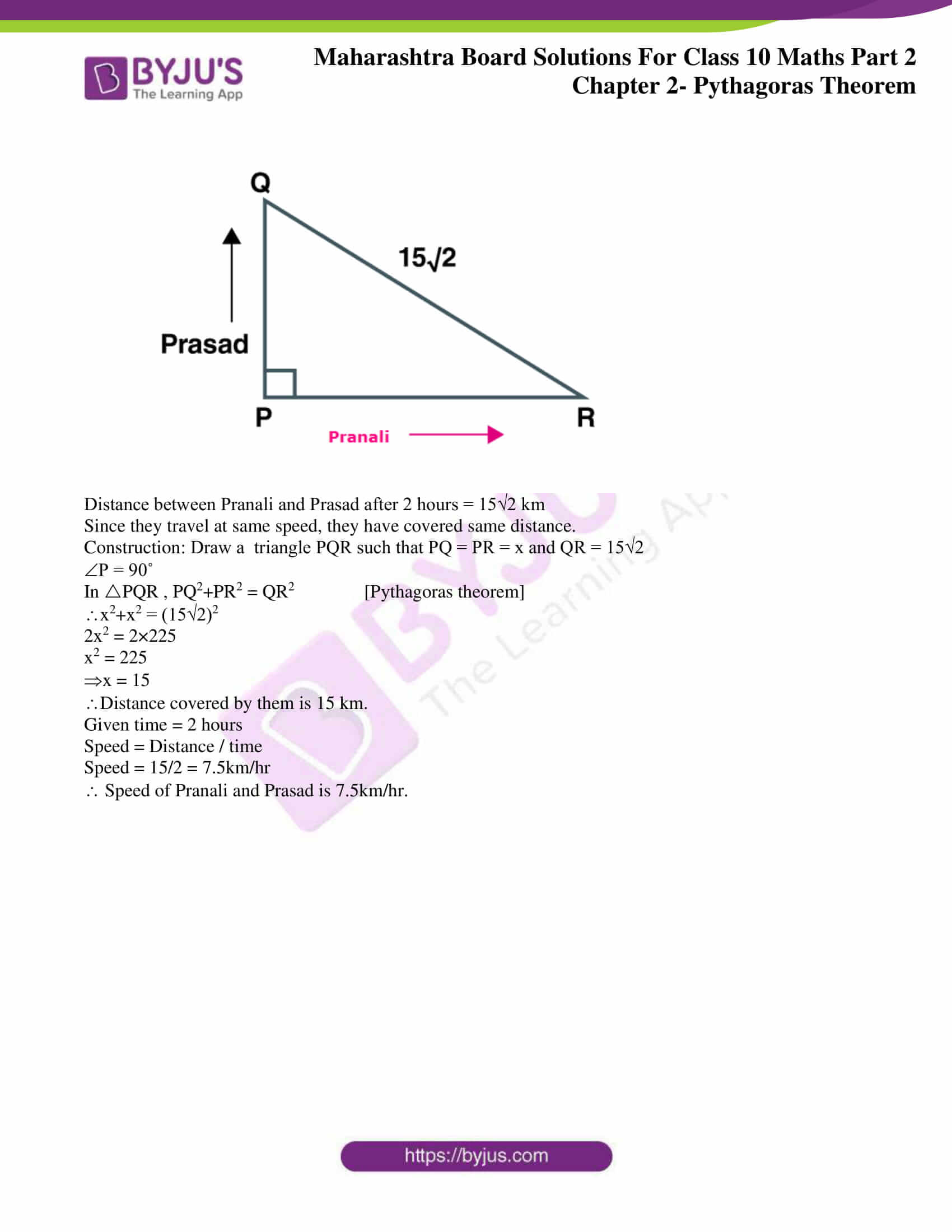### Access answers to Maths MSBSHSE Solutions For SSC Part 2 Chapter 2 – Pythagoras Theorem

Practice set 2.1 Page 38

1. Identify, with reason, which of the following are Pythagorean triplets.

(i)(3, 5, 4)

(ii)(4, 9, 12)

(iii)(5, 12, 13)

(iv) (24, 70, 74)

(v)(10, 24, 27)

(vi)(11, 60, 61)

Solution:

(i)(3, 5, 4)

32 = 9

42 = 16

52 = 25

Here 9+16 = 25

52 = 32+42

The square of largest number is equal to sum of squares of the other two numbers.

(3, 5, 4) is a Pythagorean triplet.

(ii)(4, 9, 12)

42 = 16

92 = 81

122 = 144

Here 42+92 ≠ 122

The square of largest number is not equal to sum of squares of the other two numbers.

(4, 9, 12) is not a Pythagorean triplet.

(iii)(5, 12, 13)

52 = 25

122 = 144

132 = 169

Here 52+ 122 = 132

The square of largest number is equal to sum of squares of the other two numbers.

(5, 12, 13) is a Pythagorean triplet.

(iv) (24, 70, 74)

242 = 576

702 = 4900

742 = 5476

Here 242+ 702 = 742

The square of largest number is equal to sum of squares of the other two numbers.

(24, 70, 74)is a Pythagorean triplet.

(v)(10, 24, 27)

102 = 100

242 = 576

272 = 729

Here 102+242 ≠ 272

The square of largest number is not equal to sum of squares of the other two numbers.

(10, 24, 27) is not a Pythagorean triplet.

(vi)(11, 60, 61)

112 = 121

602 = 3600

612 = 3721

Here 112+602 = 612

The square of largest number is equal to sum of squares of the other two numbers.

(11, 60, 61)is a Pythagorean triplet.

2. In figure 2.17, MNP = 90°, seg NQ seg MP, MQ = 9, QP = 4, find NQ.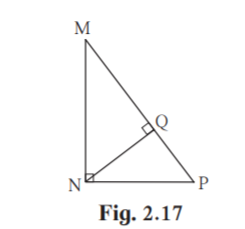Solution:

In MNP , MNP = 90˚, seg NQ ⊥ seg MP

MQ = 9 , QP = 4 [Given]

NQ = √(MQ×QP) [Theorem of geometric mean]

NQ = √(9×4) = √36 = 6

Hence NQ = 6 units.

3. In figure 2.18, QPR = 90°, seg PM seg QR and Q-M-R, PM = 10, QM = 8, find QR.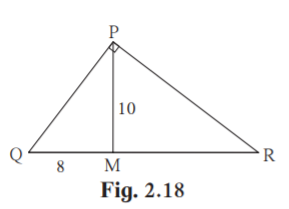Solution:

In PQR , QPR = 90˚

seg PM ⊥ seg QR [Given]

PM2 = QM×MR [Theorem of geometric mean]

102 = 8×MR

MR = 100/8 = 12.5

QR = QM + MR

QR = 8+12.5 = 20.5

Hence measure of QR is 20.5 units.

4. See figure 2.19. Find RP and PS using the information given in PSR.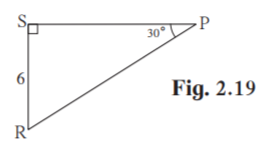Solution:

In PSR , P = 30˚ , S = 90˚

R = 180 – (90+30) = 60˚ [Angle Sum property of triangle]

PSR is a 30˚ – 60˚ – 90˚ triangle.

SR = (½)×PR [side opposite to 30˚]

6 = (½)× PR

PR = 12

PS = √(PR2 -SR2 [Pythagoras theorem]

PS = √(122-62)

PS = √(144-36)

PS = √108

PS = 6√3

Hence RP = 12 units and PS = 6√3 units.

5. For finding AB and BC with the help of information given in figure 2.20, complete following activity.

AB = BC ….____

BAC = _____

AB = BC = ____× AC

= ____ ×√8

= ____ × 2√2

= ____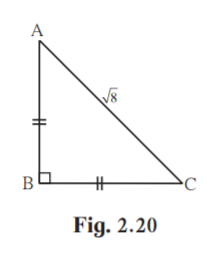Solution:

AB = AC [Given ]

BAC = BCA [Angles opposite to equal sides of an isosceles triangle are equal]

AB = BC = 1/√2× AC [By 45˚ – 45˚-90˚ theorem]

=(1/√2) ×√8

=(1/√2) × 2√2

= 2

Practice set 2.2 Page 43

1. In PQR, point S is the midpoint of side QR. If PQ = 11,PR = 17, PS =13, find QR.

Solution: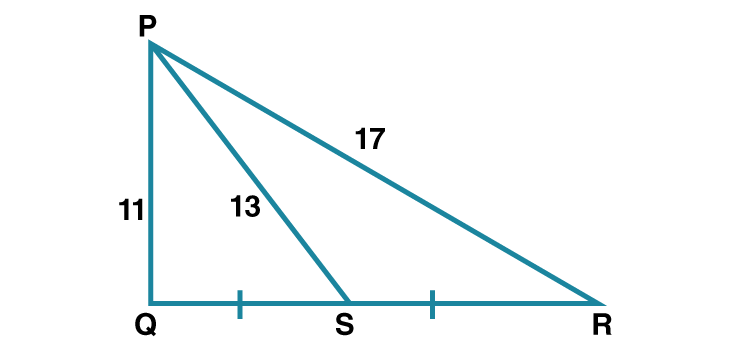Given , S is the midpoint of QR .

PS is the median.

PQ2+PR2 = 2 PS2+2SR2 [By Apollonius theorem]

112+172 = 2×132+2×SR2

121+289 = 2×169+2×SR2

2SR2 = 121+289-338

2SR2 = 72

SR2 = 72/2 = 36

SR = 6

Since S is the midpoint of QR , QR = 2SR

QR = 2×6 = 12

Hence QR = 12 units.

2. In ABC, AB = 10, AC = 7, BC = 9 then find the length of the median drawn from point C to side AB

Solution: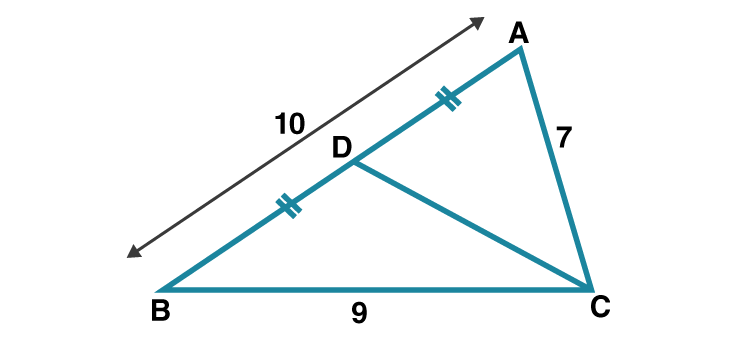Let CD is the median drawn from C to AB.

Given AB = 10

AD = (1/2)×AB [D is the midpoint of side AB]

Since CD is the median

72+92 = 2 CD2+2×52

2 CD2 = 72+92-2×52

2 CD2 = 80

CD2 = 40

Taking square roots on both sides

CD = 2√10

Hence the length of median drawn from point C to side AB is 2√10 units.

3. In the figure 2.28 seg PS is the median of PQR and PTQR. Prove that,

(1) PR2 = PS2 + QR × ST +( QR/ 2 )2

(ii) PQ2 = PS2 – QR ×ST +( QR /2)2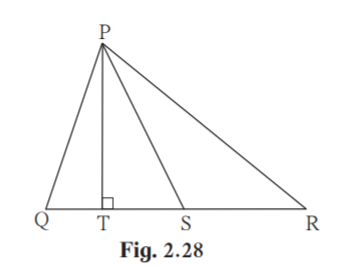Solution: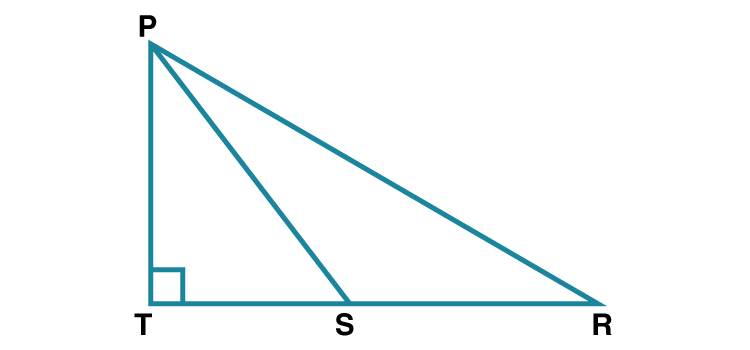(1) QS = ½ QR ……(i) [S is the midpoint of QR]

SR = ½ QR ……(ii)

QS = SR [From (i) and (ii)]

PT ⊥QR [Given]

PSR is an obtuse angle. [From figure]

PR2 = SR2+PS2+2SR×ST …..(iii) [Application of Pythagoras theorem]

Substitute SR = ½ QR in (iii)

PR2 =[(½)QR]2+PS2+2(1/2)QR×ST

PR2 =[(½)QR]2+PS2+QR×ST

PR2 = PS2 + QR×ST +(QR/ 2 )2

Hence proved.

(ii) PT⊥QS [Given]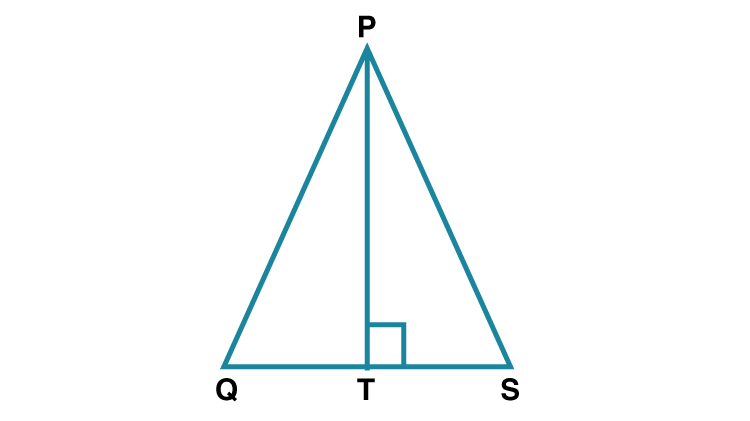PSQ is an acute angle [From figure]

PQ2 = QS2+PS2-2QS×ST [Application of Pythagoras theorem]

PR2 = [(1/2)QR]2+PS2-2(1/2 )QR×ST

PR2 = (QR/2)2+PS2-QR×ST

PR2 = PS2-QR×ST+( QR /2)2

Hence proved.

Problem set 2 Page 43

1. Some questions and their alternative answers are given. Select the correct alternative.

(1) Out of the following which is the Pythagorean triplet?

(A) (1, 5, 10)

(B) (3, 4, 5)

(C) (2, 2, 2)

(D) (5, 5, 2)

Solution:

(A) (1, 5, 10)

Here 12+52 ≠ 102

The square of largest number is not equal to sum of squares of the other two numbers.

So (1, 5, 10) is not a Pythagorean triplet.

(B) (3, 4, 5)

Here 32+42 = 52

The square of largest number is equal to sum of squares of the other two numbers.

So (3, 4, 5) is a Pythagorean triplet.

(C) (2, 2, 2)

Here 22+22 ≠ 22

The square of largest number is not equal to sum of squares of the other two numbers.

So (2, 2, 2) is not a Pythagorean triplet.

(D) (5, 5, 2)

Here 22+52 ≠ 52

The square of largest number is not equal to sum of squares of the other two numbers.

So (5, 5, 2) is not a Pythagorean triplet.

Hence option B is the correct answer.

3. In RST, S = 90°, T = 30°, RT = 12 cm then find RS and ST.

Solution: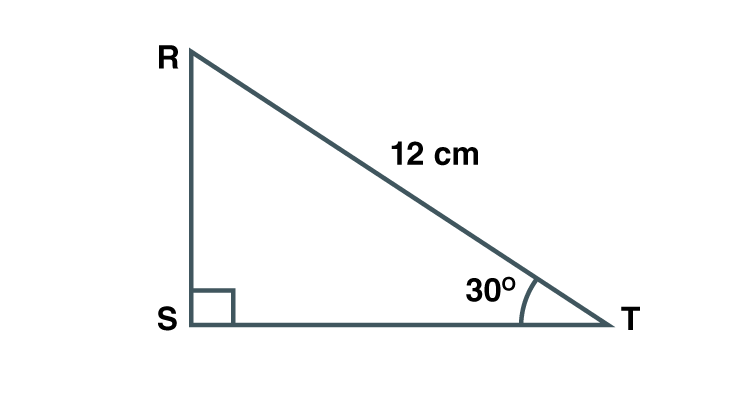Given S = 90˚ , T = 30˚

R = 180-(90+30) = 60˚ [Sum of angles of triangle is equal to 180˚]

RST is a 30˚ – 60˚- 90˚ triangle

RS = ½ RT [Side opposite to 30˚]

RS = ½ ×12 = 6

ST = √3/2 RT [side opposite to 60˚]

ST = (√3/2 )×12

ST = 6√3

Hence RS = 6 cm and ST = 6√3cm.

4. Find the diagonal of a rectangle whose length is 16 cm and area is 192 sq.cm.

Solution: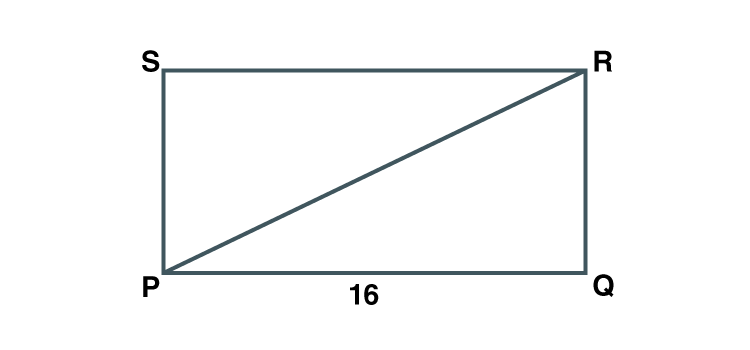Let PQRS be the rectangle.

Let length be PQ = 16 cm

Area of rectangle = Length × Breadth

Area of rectangle PQRS = PQ×QR

192 = 16×QR

QR = 192/16 = 12cm

Now in PQR , Q = 90˚ [Angles of a rectangle are 90˚]

PR2 = PQ2+QR2 [Pythagoras theorem]

PR2 = 162+122

PR2 = 256+144 = 400

PR = 20

Hence the diagonal of the rectangle is 20cm long.

5*. Find the length of the side and perimeter of an equilateral triangle whose height is √3 cm.

Solution: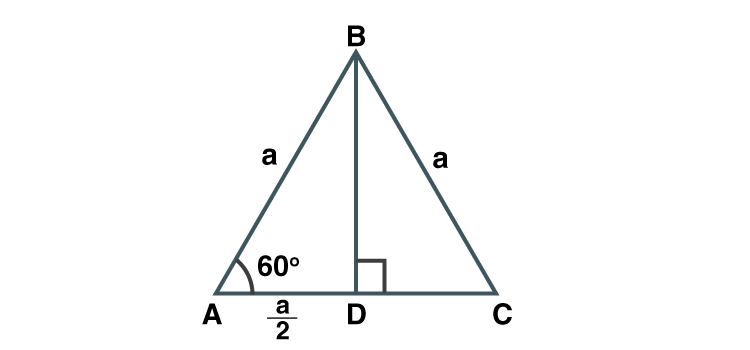Let ABC be the equilateral triangle with side a.

Let BD be height of the triangle.

Since ABC is equilateral, BD is a perpendicular bisector.

BD = √3 [given height = √3]

AB = a

Applying Pythagoras theorem in ABD

a2 = (a/2)2+(√3)2

a2 = (a2/4)+3

(3/4)a2 = 3

a2 = 4

a = 2

Hence length of side of equilateral triangle is 2 cm.

Perimeter = 3×2 = 6 [Perimeter of equilateral triangle = 3×side]

Hence perimeter of equilateral triangle is 6 cm.

6. In ABC seg AP is a median. If BC = 18, AB2 + AC2 = 260 Find AP.

Solution: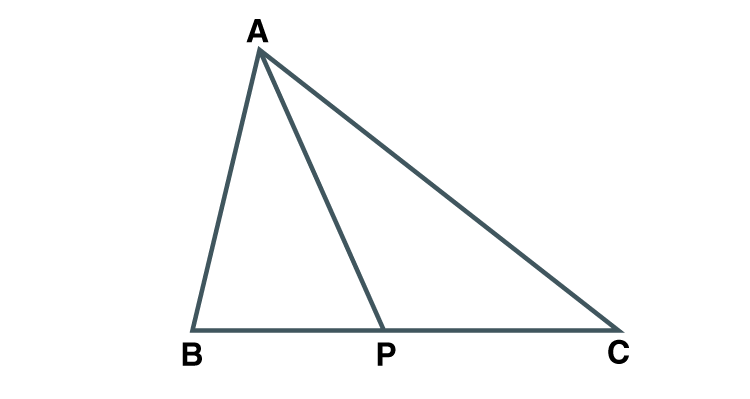Given AP is the median.

PC = BC/2

PC = 18/2 =9

AB2+AC2 = 2AP2+2PC2 [Apollonius theorem]

260 = 2AP2+2×92

2AP2 = 260-2×92

2AP2 = 260-162

AP2 = 68/2 = 49

Taking square roots on both sides

AP = 7

Hence AP = 7 units.

7*. ABC is an equilateral triangle. Point P is on base BC such that PC = 1/3 BC, if AB = 6 cm find AP.

Solution:

Given ABC is an equilateral triangle.

PC = (1/3) BC

PC = (1/3)×6 [BC = 6, side of equilateral triangle]

PC = 2

Construction: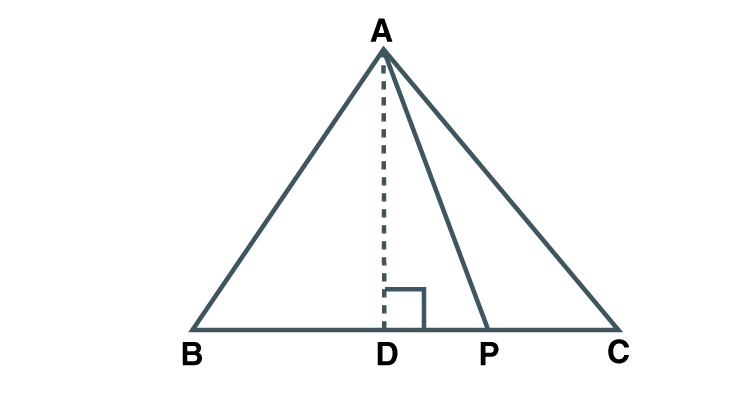D = 90˚

ADC is a 30˚- 60˚- 90˚triangle

AD = (√3/2)×AC [Side opposite to 60˚]

DC = (1/2)×6 = 3cm

DC = DP+PC [D-P-C]

3 = DP +2

DP = 1

In ADP , D = 90˚

Applying Pythagoras theorem

AP2 = (3√3)2+12

AP2 = 28

AP = 2√7 cm

Hence AP = 2√7cm.

8. From the information given in the figure 2.31, prove that PM = PN = √3×a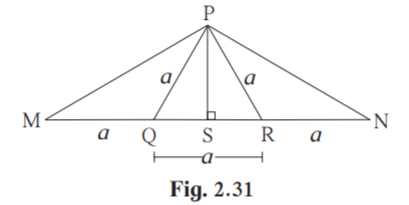Solution:

Proof: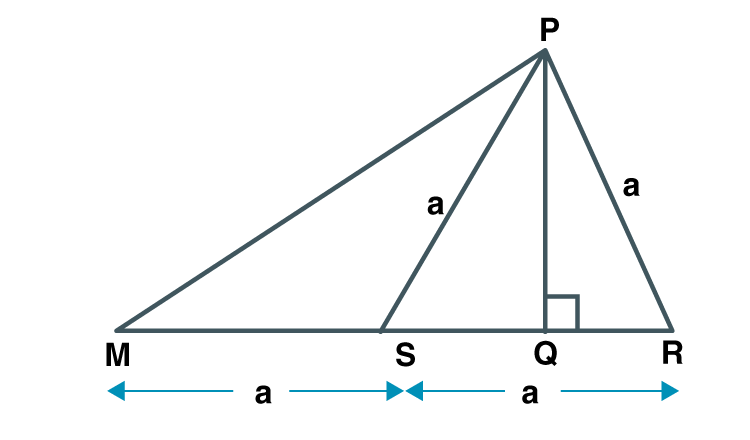In PRM

Given MQ = QR = a

Q is the midpoint of MR .

PQ is the median.

PR2+PM2 = 2PQ2+2QM2 [Apollonius theorem]

a2+PM2 = 2a2+2a2

PM2 = 3a2

PM = √3a…………(i)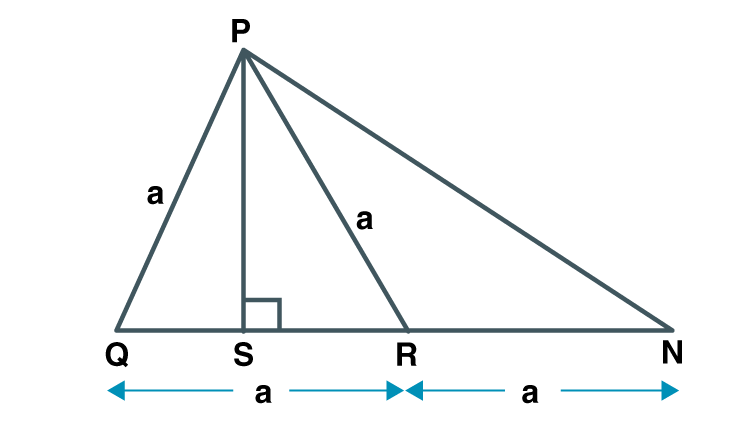In PQN

Given NR = QR = a

R is the midpoint of QN.

PR is the median.

PN2+PQ2 = 2PR2+2RN2 [Apollonius theorem]

PN2+a2 = 2a2+2a2

PN2 = 3a2

PN = √3a…………..(ii)

From (i) and (ii) PM = PN = √3×a

Hence proved.

9. Prove that the sum of the squares of the diagonals of a parallelogram is equal to the sum of the squares of its sides.

Solution:

Construction:

Draw a parallelogram ABCD. Let diagonals AC and BD meet at P.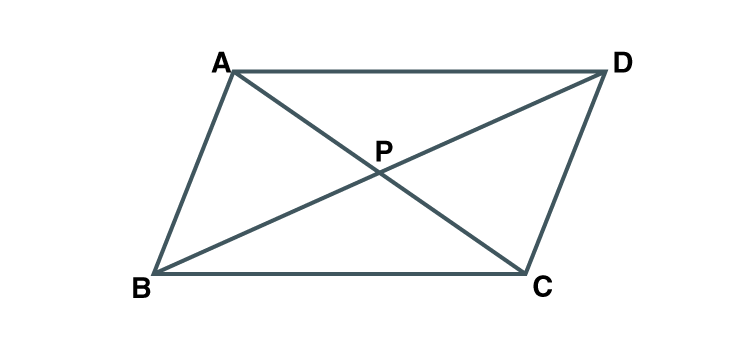To prove : AC2+BD2 = AB2+BC2+CD2+DA2

AB = CD [Opposite sides of parallelogram are equal]

BC = DA [Opposite sides of parallelogram are equal]

Since diagonals of a parallelogram bisect each other,

AP = ½ AC

BP = ½ BD

P is the midpoint of diagonals AC and BD.

InABC , BP is the median.

AB2+BC2 = 2AP2+2BP2 [Apollonius theorem]

AB2+BC2 = 2[(1/2)AC]2+2[(1/2)BD]2

AB2+BC2 =AC2/2+BD2/2

2(AB2+BC2) = AC2+BD2

2AB2+2BC2 = AC2+BD2

AB2+ AB2+ BC2+BC2 = AC2+BD2

AB2+ CD2+ BC2+DA2 = AC2+BD2

AC2+BD2 = AB2+BC2+CD2+DA2

Hence proved.

10. Pranali and Prasad started walking to the East and to the North respectively, from the same point and at the same speed. After 2 hours distance between them was 15√2 km. Find their speed per hour.

Solution: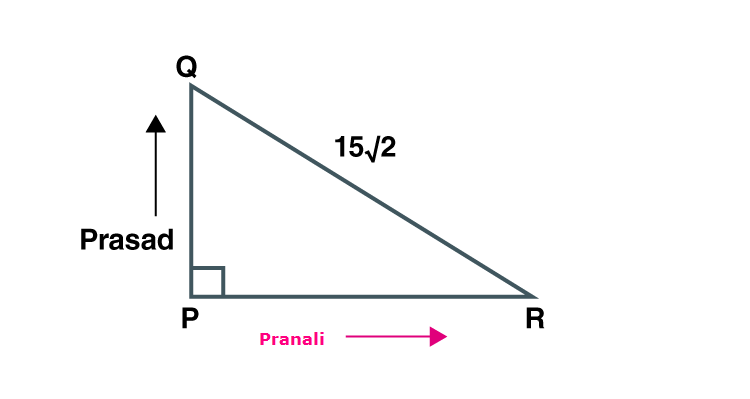Distance between Pranali and Prasad after 2 hours = 15√2 km

Since they travel at same speed, they have covered same distance.

Construction: Draw a triangle PQR such that PQ = PR = x and QR = 15√2

P = 90˚

In PQR , PQ2+PR2 = QR2 [Pythagoras theorem]

x2+x2 = (15√2)2

2x2 = 2×225

x2 = 225

x = 15

Distance covered by them is 15 km.

Given time = 2 hours

Speed = Distance / time

Speed = 15/2 = 7.5km/hr

Speed of Pranali and Prasad is 7.5km/hr.

Pythagoras theorem is the fundamental theorem in Mathematics, which defines the relation between the hypotenuse, base and altitude of a right angled triangle. According to this theorem, the square of hypotenuse is equal to the sum of squares of altitude and base of a right angled triangle. Apollonius’ theorem is also explained in this chapter. Follow BYJU’S to gain more information on mathematical concepts and learn Pythagoras Theorem and solutions.

## Frequently Asked Questions on Maharashtra State Board Solutions for Class 10 Maths Part 2 Chapter 2 Pythagorus Theorem

### Why are these Maharashtra State Board Class 10 Maths Part 2 Chapter 2 Solutions important?

These solutions are devised in such a way that  designed in a way that every student can easily grasp the concept into their minds and clarify whatever doubts they have about the topic. The solutions provided are self-explanatory and the step by step guidance will help the students. Also, the board exam aspirants can assess their learning abilities and exam preparations with these solutions.